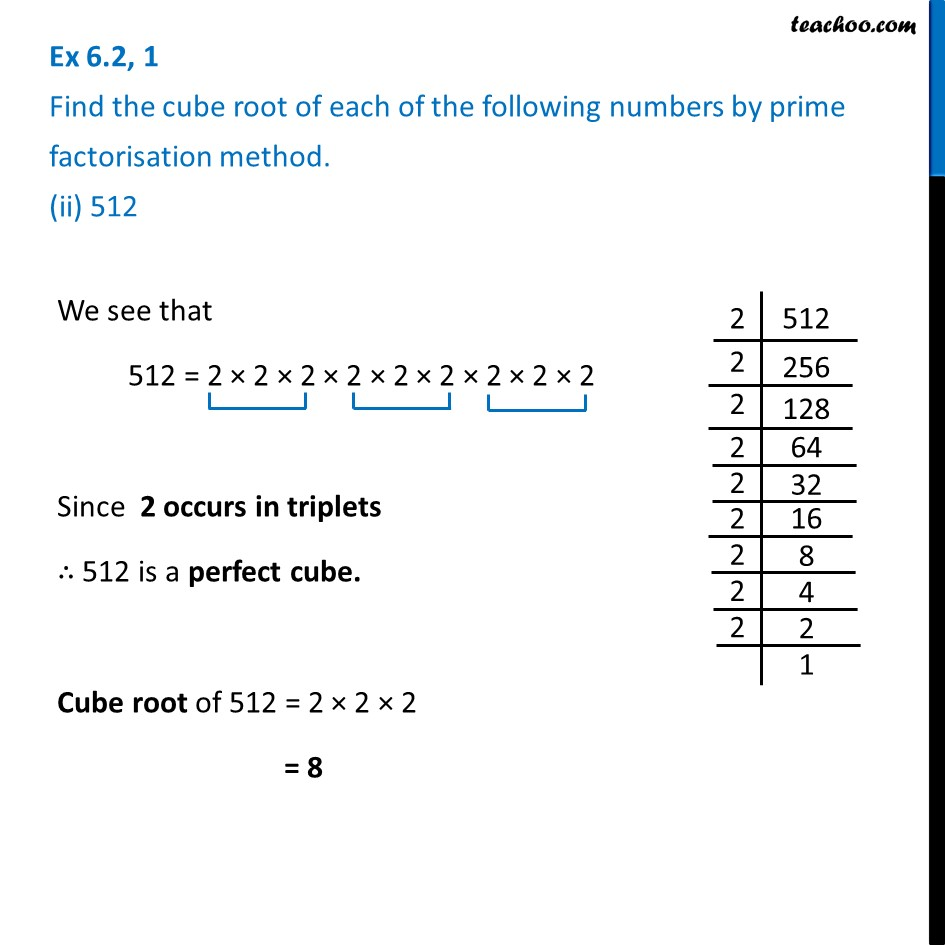Ex 6.2

Chapter 6 Class 8 Cubes and Cube Roots
Serial order wiseLearn in your speed, with individual attention - Teachoo Maths 1-on-1 Class

### Transcript

Ex 6.2, 1 Find the cube root of each of the following numbers by prime factorisation method. (ii) 512We see that 512 = 2 × 2 × 2 × 2 × 2 × 2 × 2 × 2 × 2 Since 2 occurs in triplets ∴ 512 is a perfect cube. Cube root of 512 = 2 × 2 × 2 = 8